## Wizard Recommends

I don’t understand how it is that in your Texas Hold ’Em power tables A-7 ranks lower than KJ suited but on your calculator A7 has higher probabilty of winning.

Francisco

Good question. For the benefit of other readers, here are the odds against a random hand with each of those two starting hands in a two-player game:

### K-J Suited vs. A-7 Off-Suit

 Hand Win Lose Draw Expected Value K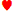J0.6148 0.3634 0.0218 0.2513 A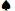7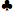0.5717 0.3949 0.0334 0.1768

However, according to my Two-Player Texas Hold ’em Calculator, the probabilities of these two hands facing each other are:

A7 wins = 53.52%
KJ wins = 46.10%
Tie = 0.39%

So KJ suited is ranked higher in my table, but inferior to the A7 unsuited head to head. Why?

The answer is hard to explain. With two hands going against each other, you have to consider how they interact with each other. For example, in the table of starting hand strength, AK off-suit is only slightly better than AQ off-suit, with expected values of .3064 and .2886 respectively. However, if you pit them against each other, the AK crushes the AQ, as follows:

AK wins = 71.72%
AQ wins = 23.69%
Tie = 4.58%

In the A7-offsuit vs. KJ-suited, the ace towers over the king and jack. The greatest chance of the KJ player winning is if he pairs up the K or J and no aces appear. I show the probability of that is 37.73% only. The rest of the 46.10% probability of the KJ winning comes from higher hands.

While A7 is strong against KJ, it is more often dominated than KJ by random hands.

Maybe this is a bad comparison, but it is kind of like the game rock paper scissors. Among skilled players, individually each throw should have about the same power rating. However, if one player throws paper and the other scissors, the power ratings mean nothing, and scissors will win.

At the Bighorn and Longhorn in Las Vegas, they allow doubling on three cards in blackjack. Are there any strategy changes I should make under this rule?

Dr. Baker from Walnut Grove, MN

A reader of my Wizard of Vegas site says the following changes should be made, relative to the standard multi-deck strategy where the dealer hits a soft 17:

• Hit soft 13 vs. 5 or 6
• Hit 2-card soft 15 vs. 4
• Hit 3,3 vs. 2

The reason for hitting the soft hands is you might get a better soft double after the hit. The value of hitting the threes is higher under this rule, because getting an ace would be a good 3-card double.

This question was raised and discussed in the forum of my companion site Wizard of Vegas.

If a coin is flipped 100 times, what is the probability of getting a streak of at least 7 heads in a row at least once?

Don from New York

If there is an easy, non-recursive, expression for the answer, I’m not aware of it. However, there is an easy recursive answer.

f(n)= pr(tails in first flip)×f(n-1) +
pr(heads in first flip, tails in second flip)×f(n-2) +
pr(heads in first 2 flips, tails in third flip)×f(n-3) +
pr(heads in first 3 flips, tails in third flip)×f(n-4) +
pr(heads in first 4 flips, tails in fourth flip)×f(n-5) +
pr(heads in first 5 flips, tails in fifth flip)×f(n-6) +
pr(heads in first 6 flips, tails in sixth flip)×f(n-7) +
pr(heads in first 7 flips) =

(1/2)×f(n-1) +
(1/2)2×f(n-2) +
(1/2)3×f(n-3) +
(1/2)4×f(n-4) +
(1/2)5×f(n-5) +
(1/2)6×f(n-6) +
(1/2)7×f(n-7) +
(1/2)7

Where:
f(n)=probability of success within n flips.
pr(x)=probability of x happening.

Spreadsheets are perfect for problems like this. In the screenshots of the spreadsheet below, I put in a probability of 0 for cells B2 to B8, because you can’t have 7 heads in a row in 6 or fewer flips. For cell B9, I put in the formula:

=(1/2)*B8+(1/2)^2*B7+(1/2)^3*B6+(1/2)^4*B5+(1/2)^5*B4+(1/2)^6*B3+(1/2)^7*B2+(1/2)^7

Then I copied and pasted it from cells B10 to cell B102, which corresponds to 100 flips. That probability is 0.317520. A random simulation confirms it.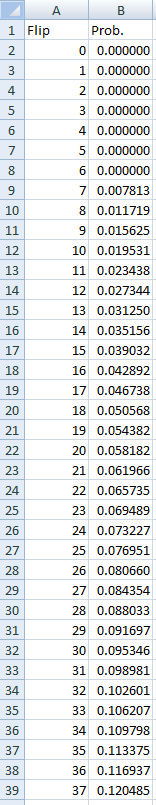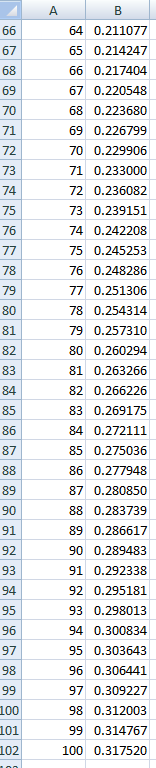By the way, in case you were wondering, the probability for at least one streak of 7 or more of heads or tails is 54.23%. The probability of one or more streaks of exactly seven heads is 17.29%.

After this originally published, Rick Percy shared his matrix algebra solution with me. Here it is in my own words. I assume the reader knows the basics of matrix albegra already.

First, there are eight possible states the flipper can be in at any one point:

p1 = Probability of success, given that you need 7 more heads from the current point.
p2 = Probability of success, given that you need 6 more heads from the current point.
p3 = Probability of success, given that you need 5 more heads from the current point.
p4 = Probability of success, given that you need 4 more heads from the current point.
p5 = Probability of success, given that you need 3 more heads from the current point.
p6 = Probability of success, given that you need 2 more heads from the current point.
p7 = Probability of success, given that you need 1 more heads from the current point.
p8 = Probability of success, given that you don’t need any more heads = 1.

Let’s define the maxtrix Sn as the probability of being in each state after the nth flip. S0 represents the probabilities before the first flip, where there is a 100% chance of being in state 0. So S0 =

` | 1 0 0 0 0 0 0 0 | `

Let T be the transformation matrix from two consecutive flips, or Sn to Sn+1, where Sn+1 = T × Sn

• If you’re in state 1 then after one flip you have a 0.5 chance of being in state 2 (with a heads), and 0.5 of remaining at state 1 (with a tails).
• If you’re in state 2 then after one flip you have a 0.5 chance of being in state 3 (with a heads), and 0.5 of returning to state 1 (with a tails).
• If you’re in state 3 then after one flip you have a 0.5 chance of being in state 4 (with a heads), and 0.5 of returning to state 1 (with a tails).
• If you’re in state 4 then after one flip you have a 0.5 chance of being in state 5 (with a heads), and 0.5 of returning to state 1 (with a tails).
• If you’re in state 5 then after one flip you have a 0.5 chance of being in state 6 (with a heads), and 0.5 of returning to state 1 (with a tails).
• If you’re in state 6 then after one flip you have a 0.5 chance of being in state 7 (with a heads), and 0.5 of returning to state 1 (with a tails).
• If you’re in state 7 then after one flip you have a 0.5 chance of being in state 8 (with a heads), and 0.5 of returning to state 1 (with a tails).
• If you’re in state 8 then you have achieved success, and will remain in state 8 with a probability of 1.0.

Putting all that in the form of the transition matrix T =

| 0.5 0.5 0.0 0.0 0.0 0.0 0.0 0.0 0.0 |
| 0.5 0.0 0.5 0.0 0.0 0.0 0.0 0.0 0.0 |
| 0.5 0.0 0.0 0.5 0.0 0.0 0.0 0.0 0.0 |
| 0.5 0.0 0.0 0.0 0.5 0.0 0.0 0.0 0.0 |
| 0.5 0.0 0.0 0.0 0.0 0.5 0.0 0.0 0.0 |
| 0.5 0.0 0.0 0.0 0.0 0.0 0.5 0.0 0.0 |
| 0.5 0.0 0.0 0.0 0.0 0.0 0.0 0.5 0.0 |
| 0.5 0.0 0.0 0.0 0.0 0.0 0.0 0.0 0.5 |
| 0.0 0.0 0.0 0.0 0.0 0.0 0.0 0.0 1.0 |

To get to the probability of each state after one flip...

(1) S1 = S0 × T

(2) S2 = S1 × T

Let’s substitute equation (1) in equation (2)...

(3) S2 = S0 × T × T = S0 × T2

(4) S3 = S2 × T

Substituting equation (3) into (4)...

(5) S3 = S0 × T2 × T = S0 × T3

We can keep doing this all the way to the state after the 100th flip...

S100 = S0 × T100

So, what is T100? Before computers it must have been a huge pain to figure such things out. However, with Excel’s MMULT function and a lot of copying and pasting we find T100 =

| 0.342616 0.171999 0.086347 0.043347 0.021761 0.010924 0.005484 0.317520 |
| 0.339863 0.170617 0.085653 0.042999 0.021586 0.010837 0.005440 0.323005 |
| 0.334379 0.167864 0.084271 0.042305 0.021238 0.010662 0.005352 0.333929 |
| 0.323454 0.162380 0.081517 0.040923 0.020544 0.010313 0.005178 0.355690 |
| 0.301693 0.151455 0.076033 0.038170 0.019162 0.009620 0.004829 0.399038 |
| 0.258346 0.129694 0.065109 0.032686 0.016409 0.008237 0.004135 0.485384 |
| 0.171999 0.086347 0.043347 0.021761 0.010924 0.005484 0.002753 0.657384 |
| 0.000000 0.000000 0.000000 0.000000 0.000000 0.000000 0.000000 1.000000 |

The term in the upper right shows us the probability of being in state 8 after 100 flips, which is 0.317520.

Recently, the Tuscany casino ran a promotion where if you got 30 blackjacks in a 30-day period, you would win a \$100 bonus. At first, the minimum bet was \$5 to get your card stamped. However, I later heard the minimum for a stamp was raised to \$15. I wrote a letter of complaint about it to the casino manager, stating in part:

I just wanted to express my disappointment in this change, if it is true. I never had a chance to take advantage of the promotion and doubt I will be able to now. The amount of time necessary to receive 30 blackjacks (I’m told about 8 hours of continuous play) seems unreasonable at \$15/hand when the promotion still offers only \$100.